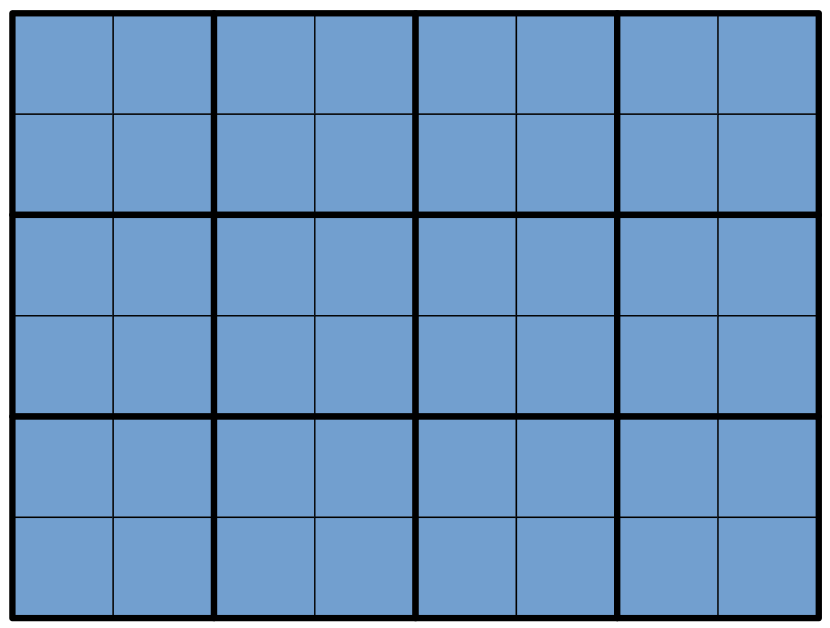## Fill The Grid Codechef Solution | GRIDBL

You have a grid with rows and columns. You have two types of tiles — one of dimensions and the other of dimensions . You want to cover the grid using these two types of tiles in such a way that:

• Each cell of the grid is covered by exactly one tile; and
• The number of tiles used is minimized.

Find the minimum number of tiles you have to use to fill the grid.

### Input Format

• The first line of input will contain a single integer , denoting the number of test cases.
• Each test case consists of a single line containing two space-separated integers

### Output Format

For each test case, print on a new line the minimum number of tiles needed to fill the grid.

### Sample Input 1

4
1 1
4 5
6 8
3 2


### Sample Output 1

1
4
0
2


### Explanation

Test case : There is only one square in the grid, and it must be filled with a single tile.

Test case : One way of tiling the grid using tiles exactly times is as follows:Test case : One way of tiling the grid using no tiles is as follows:Test case : One way of tiling the grid using tiles exactly twice is:#include <bits/stdc++.h>

using namespace std;

int main()
{

int t;
cin >> t;
while (t--)
{
int c, d;
cin >> c >> d;

if ((c % 2 == 0) && (d % 2 == 0))
{
cout << 0;
}
else if ((c % 2 != 0) && (d % 2 == 0))
{
cout << d;
}
else if ((c % 2 == 0) && (d % 2 != 0))
{
cout << c;
}
else
{
cout << c + d - 1;
}
cout << endl;
}
return 0;
}


				
/* package codechef; // don't place package name! */

import java.util.*;
import java.lang.*;
import java.io.*;

/* Name of the class has to be "Main" only if the class is public. */
class Codechef
{
public static void main (String[] args) throws java.lang.Exception
{

Scanner sc=new Scanner(System.in);
int t=sc.nextInt();
while(t-->0){
int n=sc.nextInt();
int m=sc.nextInt();

if(n%2==0){

if( m%2==0){
System.out.println(0);
}
else{
System.out.println(n);
}
}
else if(m%2==0){
System.out.println(m);
}
else{
int res=n*m - (n-1)*(m-1);
System.out.println(res);
}
}